# Monacor

Monacor develops and produces products for HiFi, Car HiFi, PA, Multimedia und Home Cinema. nacor

## no longer sold## Monacor MPT-100

order no. mo-118790

- not available (_)

Piezo tweeter.

• power handling (continuous/programme) = 75 W
• frequency range = 3500-18000 Hz
• sound pressure level SPL = 95 dB (2,83V; 1m)
• mounting diameter d = 70 mm
• overall diameter d = 98 mm
• mounting depth (not countersunk) t = 65 mm
• mass m = 0,045 kg

## Monacor MHD-182/RD

order no. mo-104450

- not available (_)

44,5 mm tweeter with high quality cone.

• power handling (continuous/programme) = 65/130 W
• frequency range = 3750-20000 Hz
• resonance frequency fs = 2500 Hz
• impedance R = 8 Ohm
• sound pressure level SPL = 103 dB (2,83V; 1m)
• voice coil diameter = 44,5 mm
• mounting diameter d = 91 mm
• overall diameter d = 101x101 mm
• mounting depth (not countersunk) t = 75 mm
• mass m = 1,6 kg

## Monacor MRD-90N

order no. mo-104200

- not available (_)

25 mm driver with high quality cone.

• power handling (continuous/programme) = 30/75 W
• frequency range = 3450-20000 Hz
• resonance frequency fs = 2300 Hz
• impedance R = 8 Ohm
• sound pressure level SPL = 104 dB (2,83V; 1m)
• voice coil diameter = 25 mm
• overall diameter d = 65x45 mm
• mass m = 0,15 kg

## Monacor HT-95PA

order no. mo-102080

- not available (_)

25,4 mm tweeter with high quality cone.

• power handling (continuous/programme) = 30/60 W
• frequency range = 3300-20000 Hz
• resonance frequency fs = 2200 Hz
• impedance R = 4 Ohm
• sound pressure level SPL = 101 dB (2,83V; 1m)
• voice coil diameter = 25,4 mm
• mounting diameter d = 72 mm
• overall diameter d = 97 mm
• mounting depth (not countersunk) t = 45 mm
• mass m = 0,55 kg## Monacor MHD-120

order no. mo-100230

- not available (_)

.

• power handling (continuous/programme) = 50/100 W
• frequency range = 2250-18000 Hz
• resonance frequency fs = 1500 Hz
• impedance R = 8 Ohm
• sound pressure level SPL = 105 dB (2,83V; 1m)
• voice coil diameter = 24,5 mm## Monacor CDX1-1745

order no. mo-105030

- not available (_)

.

• power handling (continuous/programme) = 75/180 W
• frequency range = 1950-18500 Hz
• resonance frequency fs = 1300 Hz
• impedance R = 8 Ohm
• sound pressure level SPL = 112 dB (2,83V; 1m)
• voice coil diameter = 44 mm
• overall diameter d = 120 mm
• mounting depth (not countersunk) t = 55 mm## Monacor MHD-172

order no. mo-104440

- not available (_)

.

• power handling (continuous/programme) = 50/100 W
• frequency range = 1800-22000 Hz
• resonance frequency fs = 1200 Hz
• impedance R = 8 Ohm
• sound pressure level SPL = 102 dB (2,83V; 1m)
• voice coil diameter = 34,5 mm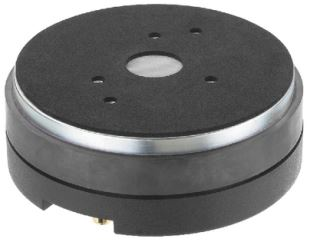## Monacor MRD-180

order no. mo-104380

- not available (_)

.

• power handling (continuous/programme) = 80/180 W
• frequency range = 1200-19000 Hz
• resonance frequency fs = 800 Hz
• impedance R = 8 Ohm
• sound pressure level SPL = 109 dB (2,83V; 1m)
• voice coil diameter = 44,4 mm
• overall diameter d = 134 mm
• mounting depth (not countersunk) t = 58 mm## Monacor MRD-190N

order no. mo-105090

- not available (_)

.

• power handling (continuous/programme) = 75/150 W
• frequency range = 1200-20000 Hz
• resonance frequency fs = 800 Hz
• impedance R = 8 Ohm
• sound pressure level SPL = 112 dB (2,83V; 1m)
• voice coil diameter = 44 mm
• overall diameter d = 100 mm
• mounting depth (not countersunk) t = 60 mm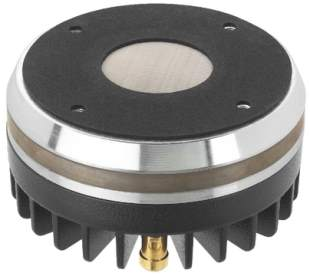## Monacor MRD-480N

order no. mo-104390

- not available (_)

. The magnet system is based on Neodymium technology.

• power handling (continuous/programme) = 125/250 W
• frequency range = 1020-16000 Hz
• resonance frequency fs = 680 Hz
• impedance R = 8 Ohm
• sound pressure level SPL = 110 dB (2,83V; 1m)
• voice coil diameter = 72 mm
• overall diameter d = 136 mm
• mounting depth (not countersunk) t = 68 mm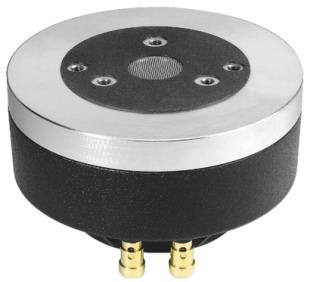## Monacor MRD-200

order no. mo-103470

- not available (_)

.

• power handling (continuous/programme) = 50/120 W
• frequency range = 840-16000 Hz
• resonance frequency fs = 560 Hz
• impedance R = 8 Ohm
• sound pressure level SPL = 110 dB (2,83V; 1m)
• voice coil diameter = 44,4 mm
• overall diameter d = 143 mm
• mounting depth (not countersunk) t = 80 mm## Monacor MRD-140

order no. mo-103460

- not available (_)

.

• power handling (continuous/programme) = 40/100 W
• frequency range = 750-16000 Hz
• resonance frequency fs = 500 Hz
• impedance R = 8 Ohm
• sound pressure level SPL = 106 dB (2,83V; 1m)
• voice coil diameter = 34,4 mm
• overall diameter d = 110 mm
• mounting depth (not countersunk) t = 63 mmrecommended cabinet 1:
closed cabinet with 6,9 L volume
from 150/94 Hz (-3dB/-8dB)

recommended cabinet 2:
28 L volume bass reflex cabinet
with 4x HP100 reflex tube, 41 cm long.
from 68/58 Hz (-3dB/-8dB).

## Monacor SP-20A/150PAM

order no. mo-103720

- not available (_)

20 cm midrange driver with high quality cone.

• power handling (continuous/programme) = 150/300 W
• resonance frequency fs = 98 Hz
• impedance R = 8 Ohm
• sound pressure level SPL = 98 dB (2,83V; 1m)
• DC resistance Re = 5,3 Ohm
• force factor BL = 9,8 N/A
• voice coil inductance L = 0,5 mH
• effective piston radiating area Sd = 225 cm2
• effective mechanical mass incl. air load mms = 16,5 g
• equivalent volume of compliance Vas = 11 l
• total Q factor Qts = 0,52 (Qms=4,58, Qes=0,59)
• voice coil diameter = 38 mm
• maximum peak linear excursion vibration xlin = +/- 1 mm
• mounting diameter d = 188 mm
• overall diameter d = 206 mm
• mounting depth (not countersunk) t = 80,7 mm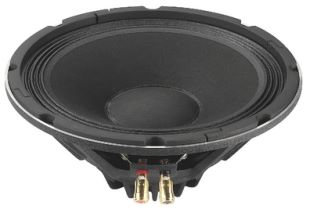## Monacor SP-10A/250NEO

order no. mo-104600

- not available (_)

25 cm bass-midrange driver with high quality cone. The magnet system is based on Neodymium technology. The basket is made of die-cast.

• power handling (continuous/programme) = 250/500 W
• frequency range = 30-3000 Hz
• resonance frequency fs = 80 Hz
• impedance R = 8 Ohm
• sound pressure level SPL = 99 dB (2,83V; 1m)
• DC resistance Re = 5,7 Ohm
• force factor BL = 12,01 N/A
• voice coil inductance L = 0,3 mH
• effective piston radiating area Sd = 347 cm2
• effective mechanical mass incl. air load mms = 32 g
• equivalent volume of compliance Vas = 19 l
• total Q factor Qts = 0,63 (Qms=16,59, Qes=0,66)
• voice coil diameter = 50 mm
• maximum peak linear excursion vibration xlin = +/- 3 mm
• mounting diameter d = 234 mm
• overall diameter d = 260 mm
• mounting depth (not countersunk) t = 110 mmrecommended cabinet 1:
closed cabinet with 7,7 L volume
from 137/87 Hz (-3dB/-8dB)

recommended cabinet 2:
28 L volume bass reflex cabinet
with HP100 reflex tube, 10 cm long.
from 70/55 Hz (-3dB/-8dB).

## Monacor SP-10/100PA

order no. mo-102490

- not available (_)

25 cm midrange driver with high quality cone.

• power handling (continuous/programme) = 100/200 W
• frequency range = 70-5000 Hz
• resonance frequency fs = 65 Hz
• impedance R = 8 Ohm
• sound pressure level SPL = 98 dB (2,83V; 1m)
• DC resistance Re = 6,9 Ohm
• force factor BL = 14,4 N/A
• voice coil inductance L = 0,75 mH
• effective piston radiating area Sd = 350 cm2
• effective mechanical mass incl. air load mms = 31 g
• equivalent volume of compliance Vas = 32 l
• total Q factor Qts = 0,38 (Qms=4,1, Qes=0,42)
• voice coil diameter = 50 mm
• maximum peak linear excursion vibration xlin = +/- 2,5 mm
• mounting diameter d = 234 mm
• overall diameter d = 258 mm
• mounting depth (not countersunk) t = 106 mmrecommended cabinet 1:
closed cabinet with 4 L volume
from 170/108 Hz (-3dB/-8dB)

recommended cabinet 2:
11 L volume bass reflex cabinet
with HP100 reflex tube, 21 cm long.
from 97/74 Hz (-3dB/-8dB).

## Monacor SP-10/150PA

order no. mo-102500

- not available (_)

24 cm midrange driver with high quality cone.

• power handling (continuous/programme) = 150/300 W
• frequency range = 60-5000 Hz
• resonance frequency fs = 60 Hz
• impedance R = 8 Ohm
• sound pressure level SPL = 98 dB (2,83V; 1m)
• DC resistance Re = 6,9 Ohm
• force factor BL = 18,2 N/A
• voice coil inductance L = 0,94 mH
• effective piston radiating area Sd = 350 cm2
• effective mechanical mass incl. air load mms = 38 g
• equivalent volume of compliance Vas = 34 l
• total Q factor Qts = 0,28 (Qms=5,36, Qes=0,3)
• voice coil diameter = 65 mm
• maximum peak linear excursion vibration xlin = +/- 4 mm
• mounting diameter d = 228 mm
• overall diameter d = 258 mm
• mounting depth (not countersunk) t = 118 mmrecommended cabinet 1:
closed cabinet with 12,8 L volume
from 115/73 Hz (-3dB/-8dB)

recommended cabinet 2:
49 L volume bass reflex cabinet
with HP100 reflex tube, 6 cm long.
from 56/46 Hz (-3dB/-8dB).

## Monacor SPA-25PA

order no. mo-104030

- not available (_)

25 cm midrange driver with paper cone. The basket is made of die-cast.

• power handling (continuous/programme) = 125/250 W
• frequency range = 60-6000 Hz
• resonance frequency fs = 60 Hz
• impedance R = 8 Ohm
• sound pressure level SPL = 97 dB (2,83V; 1m)
• DC resistance Re = 5,7 Ohm
• force factor BL = 10,87 N/A
• voice coil inductance L = 0,5 mH
• effective piston radiating area Sd = 52 cm2
• effective mechanical mass incl. air load mms = 29 g
• equivalent volume of compliance Vas = 41 l
• total Q factor Qts = 0,42 (Qms=2,23, Qes=0,52)
• voice coil diameter = 50 mm
• maximum peak linear excursion vibration xlin = +/- 1,5 mm
• mounting diameter d = 235 mm
• overall diameter d = 265 mm
• mounting depth (not countersunk) t = 99 mm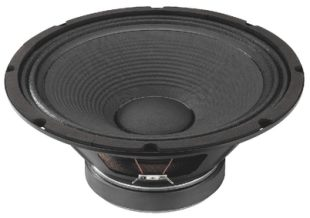recommended cabinet 1:
closed cabinet with 27 L volume
from 96/61 Hz (-3dB/-8dB)

recommended cabinet 2:
110 L volume bass reflex cabinet
with 2x HP100 reflex tube, 6 cm long.
from 46/38 Hz (-3dB/-8dB).

## Monacor SP-12/100PA

order no. mo-102510

- not available (_)

30 cm bass driver with high quality cone.

• power handling (continuous/programme) = 100/200 W
• frequency range = 60-5000 Hz
• resonance frequency fs = 55 Hz
• impedance R = 8 Ohm
• sound pressure level SPL = 100 dB (2,83V; 1m)
• DC resistance Re = 6,8 Ohm
• force factor BL = 14,1 N/A
• voice coil inductance L = 0,8 mH
• effective piston radiating area Sd = 531 cm2
• effective mechanical mass incl. air load mms = 43 g
• equivalent volume of compliance Vas = 68 l
• total Q factor Qts = 0,46 (Qms=4,34, Qes=0,51)
• voice coil diameter = 50 mm
• maximum peak linear excursion vibration xlin = +/- 2,5 mm
• mounting diameter d = 280 mm
• overall diameter d = 310 mm
• mounting depth (not countersunk) t = 125 mmrecommended cabinet 1:
closed cabinet with 6,3 L volume
from 168/106 Hz (-3dB/-8dB)

recommended cabinet 2:
15 L volume bass reflex cabinet
with HP100 reflex tube, 14 cm long.
from 100/75 Hz (-3dB/-8dB).

## Monacor SP-12A/300NEO

order no. mo-104610

- not available (_)

30 cm bass driver with high quality cone. The magnet system is based on Neodymium technology. The basket is made of die-cast.

• power handling (continuous/programme) = 300/600 W
• frequency range = 30-3500 Hz
• resonance frequency fs = 53 Hz
• impedance R = 8 Ohm
• sound pressure level SPL = 103 dB (2,83V; 1m)
• DC resistance Re = 5,5 Ohm
• force factor BL = 17,8 N/A
• voice coil inductance L = 0,3 mH
• effective piston radiating area Sd = 500 cm2
• effective mechanical mass incl. air load mms = 45 g
• equivalent volume of compliance Vas = 68 l
• total Q factor Qts = 0,25 (Qms=7,93, Qes=0,26)
• voice coil diameter = 65 mm
• maximum peak linear excursion vibration xlin = +/- 3,5 mm
• mounting diameter d = 281 mm
• overall diameter d = 312 mm
• mounting depth (not countersunk) t = 116 mm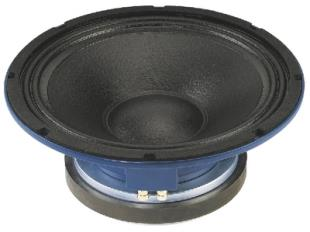recommended cabinet 1:
closed cabinet with 5,3 L volume
from 171/108 Hz (-3dB/-8dB)

recommended cabinet 2:
12,3 L volume bass reflex cabinet
with HP100 reflex tube, 18 cm long.
from 104/78 Hz (-3dB/-8dB).

## Monacor SP-30A/250PA

order no. mo-103360

- not available (_)

30 cm bass-midrange driver with high quality cone.

• power handling (continuous/programme) = 250/1000 W
• frequency range = 30-4500 Hz
• resonance frequency fs = 52 Hz
• impedance R = 8 Ohm
• sound pressure level SPL = 100 dB (2,83V; 1m)
• DC resistance Re = 5,4 Ohm
• force factor BL = 20,3 N/A
• voice coil inductance L = 0,9 mH
• effective piston radiating area Sd = 530 cm2
• effective mechanical mass incl. air load mms = 60 g
• equivalent volume of compliance Vas = 62 l
• total Q factor Qts = 0,24 (Qms=5,55, Qes=0,25)
• voice coil diameter = 76 mm
• maximum peak linear excursion vibration xlin = +/- 3,5 mm
• mounting diameter d = 283 mm
• overall diameter d = 318 mm
• mounting depth (not countersunk) t = 122 mmrecommended cabinet 1:
closed cabinet with 5,1 L volume
from 171/108 Hz (-3dB/-8dB)

recommended cabinet 2:
11,9 L volume bass reflex cabinet
with HP100 reflex tube, 19 cm long.
from 104/78 Hz (-3dB/-8dB).

## Monacor SP-30A/300NEO

order no. mo-104310

- not available (_)

30 cm bass-midrange driver with high quality cone. The magnet system is based on Neodymium technology.

• power handling (continuous/programme) = 300/600 W
• frequency range = 30-3500 Hz
• resonance frequency fs = 52 Hz
• impedance R = 8 Ohm
• sound pressure level SPL = 100 dB (2,83V; 1m)
• DC resistance Re = 5,1 Ohm
• force factor BL = 20,1 N/A
• voice coil inductance L = 1 mH
• effective piston radiating area Sd = 530 cm2
• effective mechanical mass incl. air load mms = 60 g
• equivalent volume of compliance Vas = 60 l
• total Q factor Qts = 0,24 (Qms=5,36, Qes=0,25)
• voice coil diameter = 75 mm
• maximum peak linear excursion vibration xlin = +/- 3,5 mm
• mounting diameter d = 280 mm
• overall diameter d = 314 mm
• mounting depth (not countersunk) t = 136 mmrecommended cabinet 1:
closed cabinet with 25 L volume
from 88/55 Hz (-3dB/-8dB)

recommended cabinet 2:
101 L volume bass reflex cabinet
with 2x HP100 reflex tube, 11 cm long.
from 42/35 Hz (-3dB/-8dB).

## Monacor SPA-30PA

order no. mo-104040

- not available (_)

30 cm bass-midrange driver with paper cone. The basket is made of die-cast.

• power handling (continuous/programme) = 175/350 W
• frequency range = 50-5000 Hz
• resonance frequency fs = 49 Hz
• impedance R = 8 Ohm
• sound pressure level SPL = 98 dB (2,83V; 1m)
• DC resistance Re = 6 Ohm
• force factor BL = 13,42 N/A
• voice coil inductance L = 0,87 mH
• effective piston radiating area Sd = 490 cm2
• effective mechanical mass incl. air load mms = 52 g
• equivalent volume of compliance Vas = 67 l
• total Q factor Qts = 0,45 (Qms=2,71, Qes=0,54)
• voice coil diameter = 50 mm
• maximum peak linear excursion vibration xlin = +/- 3 mm
• mounting diameter d = 284 mm
• overall diameter d = 318 mm
• mounting depth (not countersunk) t = 124 mmrecommended cabinet 1:
closed cabinet with 13,9 L volume
from 128/81 Hz (-3dB/-8dB)

recommended cabinet 2:
41 L volume bass reflex cabinet
with 2x HP100 reflex tube, 16 cm long.
from 71/54 Hz (-3dB/-8dB).

## Monacor SP-38A/300PA

order no. mo-103390

- not available (_)

38 cm bass-midrange driver with high quality cone.

• power handling (continuous/programme) = 300/1200 W
• frequency range = 30-4000 Hz
• resonance frequency fs = 48 Hz
• impedance R = 8 Ohm
• sound pressure level SPL = 102 dB (2,83V; 1m)
• DC resistance Re = 5,4 Ohm
• force factor BL = 24,1 N/A
• voice coil inductance L = 1,3 mH
• effective piston radiating area Sd = 880 cm2
• effective mechanical mass incl. air load mms = 100 g
• equivalent volume of compliance Vas = 101 l
• total Q factor Qts = 0,3 (Qms=7,45, Qes=0,31)
• voice coil diameter = 76 mm
• maximum peak linear excursion vibration xlin = +/- 4,8 mm
• mounting diameter d = 360 mm
• overall diameter d = 391 mm
• mounting depth (not countersunk) t = 162 mmopen a bigger photo

recommended cabinet 1:
closed cabinet with 24 L volume
from 86/54 Hz (-3dB/-8dB)

recommended cabinet 2:
94 L volume bass reflex cabinet
with 2x HP100 reflex tube, 14 cm long.
from 41/34 Hz (-3dB/-8dB).

## Monacor SPA-12PA

order no. mo-105280

- not available (_)

30 cm bass-midrange driver with high quality cone. The basket is made of die-cast. To avoid compression effects the driver possesses a pole piece hole.

• power handling (continuous/programme) = 150/300 W
• frequency range = f3-3500 Hz
• resonance frequency fs = 47 Hz
• impedance R = 8 Ohm
• sound pressure level SPL = 96 dB (2,83V; 1m)
• DC resistance Re = 6,3 Ohm
• force factor BL = 15,75 N/A
• voice coil inductance L = 1,1 mH
• effective piston radiating area Sd = 530 cm2
• effective mechanical mass incl. air load mms = 67 g
• equivalent volume of compliance Vas = 67 l
• total Q factor Qts = 0,43 (Qms=3,33, Qes=0,50)
• voice coil diameter = 63 mm
• maximum peak linear excursion vibration xlin = +/- 4 mm
• mounting diameter d = 282 mm
• overall diameter d = 310 mm
• mounting depth (not countersunk) t = 130 mm
• mass m = 4,5 kgrecommended cabinet 1:
closed cabinet with 20 L volume
from 109/69 Hz (-3dB/-8dB)

recommended cabinet 2:
63 L volume bass reflex cabinet
with 2x HP100 reflex tube, 13 cm long.
from 58/45 Hz (-3dB/-8dB).

## Monacor SP-30A/200PRO

order no. mo-104520

- not available (_)

29 cm bass driver with high quality cone. The basket is made of die-cast.

• power handling (continuous/programme) = 200/400 W
• frequency range = 30-3000 Hz
• resonance frequency fs = 45 Hz
• impedance R = 8 Ohm
• sound pressure level SPL = 102 dB (2,83V; 1m)
• DC resistance Re = 5,8 Ohm
• force factor BL = 13,95 N/A
• voice coil inductance L = 0,7 mH
• effective piston radiating area Sd = 530 cm2
• effective mechanical mass incl. air load mms = 43 g
• equivalent volume of compliance Vas = 114 l
• total Q factor Qts = 0,33 (Qms=4,33, Qes=0,36)
• voice coil diameter = 63 mm
• maximum peak linear excursion vibration xlin = +/- 2 mm
• mounting diameter d = 282 mm
• overall diameter d = 304 mm
• mounting depth (not countersunk) t = 127 mmrecommended cabinet 1:
closed cabinet with 24 L volume
from 113/72 Hz (-3dB/-8dB)

recommended cabinet 2:
74 L volume bass reflex cabinet
with 2x HP100 reflex tube, 8 cm long.
from 62/48 Hz (-3dB/-8dB).

## Monacor SP-38A/300NEO

order no. mo-104320

- not available (_)

37 cm bass-midrange driver with high quality cone. The magnet system is based on Neodymium technology.

• power handling (continuous/programme) = 300/600 W
• frequency range = 30-3500 Hz
• resonance frequency fs = 44 Hz
• impedance R = 8 Ohm
• sound pressure level SPL = 102 dB (2,83V; 1m)
• DC resistance Re = 5,2 Ohm
• force factor BL = 18,04 N/A
• voice coil inductance L = 0,9 mH
• effective piston radiating area Sd = 854 cm2
• effective mechanical mass incl. air load mms = 74 g
• equivalent volume of compliance Vas = 164 l
• total Q factor Qts = 0,31 (Qms=4,02, Qes=0,34)
• voice coil diameter = 75 mm
• maximum peak linear excursion vibration xlin = +/- 3,5 mm
• mounting diameter d = 353 mm
• overall diameter d = 389 mm
• mounting depth (not countersunk) t = 165 mm## Monacor SP-450PA

order no. mo-103000

- not available (_)

44 cm bass driver with high quality cone.

• power handling (continuous/programme) = 300/600 W
• frequency range = 37-3500 Hz
• resonance frequency fs = 25 Hz
• impedance R = 8 Ohm
• sound pressure level SPL = 97 dB (2,83V; 1m)
• mounting diameter d = 420 mm
• overall diameter d = 460 mm
• mounting depth (not countersunk) t = 196 mm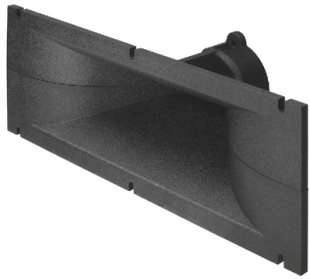## Monacor MRH-120

order no. mo-129290

- not available (_)

Horn for a compression driver with 1 inch throat.

• Throat diameter = 1 inch
• nominal angle (HxV) = 90x40 Deg
• Cut off frequency : 1500 Hz
• over all dimensions (HxWxD) = 123x365x140 mm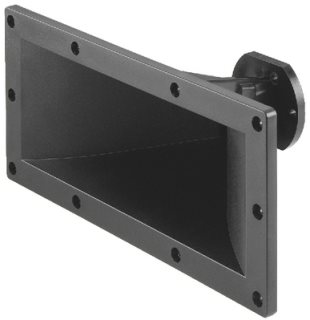## Monacor MRH-140

order no. mo-128410

- not available (_)

Horn for a compression driver with 1 inch throat.

• Throat diameter = 1 inch
• nominal angle (HxV) = 90x40 Deg
• Cut off frequency : 1700 Hz
• over all dimensions (HxWxD) = 160x360x165 mm
• baffle cutout dimensions (HxW) = 120x310 mm## Monacor MPT-196

order no. mo-110550

- not available (_)

Horn for a compression driver with 1 3/8 inch throat.

• Throat diameter = 1 3/8 inch
• nominal angle (HxV) = 100x30 Deg
• Cut off frequency : 700 Hz
• over all dimensions (HxWxD) = 130x387x160 mm
• baffle cutout dimensions (HxW) = 98x350 mm

## Monacor TRUVOX-0510

order no. mo-104950

- not available (_)

13x13 cm midrange driver with cellulose cone.

• power handling (continuous/programme) = 35/75 W
• frequency range = 300-13000 Hz
• resonance frequency fs = 110 Hz
• impedance R = 8 Ohm
• sound pressure level SPL = 93 dB (2,83V; 1m)
• DC resistance Re = 6,2 Ohm
• force factor BL = 6,2 N/A
• voice coil inductance L = 0,33 mH
• effective piston radiating area Sd = 105 cm2
• effective mechanical mass incl. air load mms = 5,5 g
• equivalent volume of compliance Vas = 2,5 l
• total Q factor Qts = 0,51 (Qms=2,0, Qes=0,68)
• voice coil diameter = 25 mm
• maximum peak linear excursion vibration xlin = +/- 1,1 mm
• mounting diameter d = 117 mm
• overall diameter d = 136x136 mm
• mounting depth (not countersunk) t = 68 mm
• mass m = 0,9 kg

## Monacor TRUVOX-0615MR

order no. mo-104960

- not available (_)

16 cm midrange driver with cellulose cone.

• power handling (continuous/programme) = 50/100 W
• frequency range = 800-5000 Hz
• resonance frequency fs = 520 Hz
• impedance R = 8 Ohm
• sound pressure level SPL = 98 dB (2,83V; 1m)
• DC resistance Re = 5,7 Ohm
• voice coil inductance L = 0,8 mH
• effective piston radiating area Sd = 169 cm2
• voice coil diameter = 38 mm
• maximum peak linear excursion vibration xlin = +/- 1,0 mm
• mounting diameter d = 144 mm
• overall diameter d = 180 mm
• mounting depth (not countersunk) t = 73 mm
• mass m = 1,4 kg

## Monacor TRUVOX-0818MR

order no. mo-104970

- not available (_)

19 cm midrange driver with cellulose cone.

• power handling (continuous/programme) = 100/200 W
• frequency range = 700-4500 Hz
• resonance frequency fs = 450 Hz
• impedance R = 8 Ohm
• sound pressure level SPL = 102 dB (2,83V; 1m)
• DC resistance Re = 6,7 Ohm
• voice coil inductance L = 0,7 mH
• effective piston radiating area Sd = 255 cm2
• voice coil diameter = 44 mm
• maximum peak linear excursion vibration xlin = +/- 1,0 mm
• mounting diameter d = 181 mm
• overall diameter d = 208 mm
• mounting depth (not countersunk) t = 83 mm
• mass m = 1,85 kg

## Monacor SP-165GI

order no. mo-102270

- not available (_)

15 cm midrange driver with high quality cone.

• power handling (continuous/programme) = 60/30 W
• frequency range = 135-8000 Hz
• resonance frequency fs = 90 Hz
• impedance R = 8 Ohm
• sound pressure level SPL = 94 dB (2,83V; 1m)
• mounting diameter d = 146 mm
• overall diameter d = 165 mm
• mounting depth (not countersunk) t = 75 mm
• mass m = 1,2 kg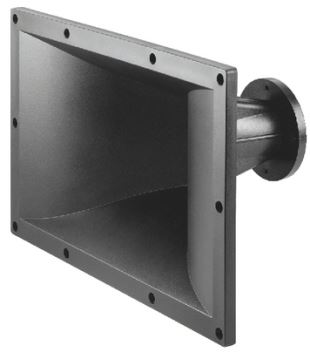## Monacor MRH-650

order no. mo-128430

- not available (_)

Horn for a compression driver with 2 inch throat.

• Throat diameter = 2 inch
• nominal angle (HxV) = 90x40 Deg
• Cut off frequency : 700 Hz
• over all dimensions (HxWxD) = 280x442x239 mm
• baffle cutout dimensions (HxW) = 235x365 mm## Monacor MRD-650

order no. mo-103480

- not available (_)

.

• power handling (continuous/programme) = 100/250 W
• resonance frequency fs = 430 Hz
• impedance R = 8 Ohm
• sound pressure level SPL = 111 dB (2,83V; 1m)
• voice coil diameter = 99 mm
• overall diameter d = 230 mm
• mounting depth (not countersunk) t = 130 mm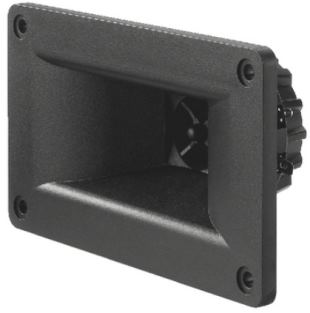## Monacor MHD-220N

order no. mo-105070

- not available (_)

.

• power handling (continuous/programme) = 30/75 W
• resonance frequency fs = 2300 Hz
• impedance R = 8 Ohm
• sound pressure level SPL = 103 dB (2,83V; 1m)
• voice coil diameter = 25 mmopen a bigger photo

recommended cabinet 1:
closed cabinet with 14 L volume
from 97/61 Hz (-3dB/-8dB)

recommended cabinet 2:
56 L volume bass reflex cabinet
with HP100 reflex tube, 10 cm long.
from 46/38 Hz (-3dB/-8dB).

## Monacor SPA-10PA

order no. mo-105270

- not available (_)

25 cm bass-midrange driver with high quality cone. The basket is made of die-cast. To avoid compression effects the driver possesses a pole piece hole.

• power handling (continuous/programme) = 125/220 W
• frequency range = 80-3500 Hz
• resonance frequency fs = 54 Hz
• impedance R = 8 Ohm
• sound pressure level SPL = 95 dB (2,83V; 1m)
• DC resistance Re = 6,2 Ohm
• force factor BL = 12,49 N/A
• voice coil inductance L = 0,9 mH
• effective piston radiating area Sd = 346 cm2
• effective mechanical mass incl. air load mms = 39 g
• equivalent volume of compliance Vas = 37 l
• total Q factor Qts = 0,45 (Qms=2,79, Qes=0,53)
• voice coil diameter = 50 mm
• maximum peak linear excursion vibration xlin = +/- 4,0 mm
• mounting diameter d = 232 mm
• overall diameter d = 262 mm
• mounting depth (not countersunk) t = 120 mm
• mass m = 3,4 kgrecommended cabinet 1:
closed cabinet with 2,5 L volume
from 171/108 Hz (-3dB/-8dB)

recommended cabinet 2:
5,7 L volume bass reflex cabinet
with HP70 reflex tube, 20 cm long.
from 104/78 Hz (-3dB/-8dB).

## Monacor SP-25A/250PA

order no. mo-103340

- not available (_)

25 cm midrange driver with high quality cone.

• power handling (continuous/programme) = 250/800 W
• resonance frequency fs = 52 Hz
• impedance R = 8 Ohm
• sound pressure level SPL = 97 dB (2,83V; 1m)
• DC resistance Re = 5,4 Ohm
• force factor BL = 19,6 N/A
• voice coil inductance L = 0,95 mH
• effective piston radiating area Sd = 350 cm2
• effective mechanical mass incl. air load mms = 55 g
• equivalent volume of compliance Vas = 29 l
• total Q factor Qts = 0,24 (Qms=6,3, Qes=0,25)
• voice coil diameter = 76 mm
• maximum peak linear excursion vibration xlin = +/- 2,5 mm
• mounting diameter d = 238 mm
• overall diameter d = 264 mm
• mounting depth (not countersunk) t = 109 mmrecommended cabinet 1:
closed cabinet with 4,9 L volume
from 158/100 Hz (-3dB/-8dB)

recommended cabinet 2:
17 L volume bass reflex cabinet
with HP100 reflex tube, 14 cm long.
from 82/65 Hz (-3dB/-8dB).

## Monacor SP-25A/200NEO

order no. mo-104300

- not available (_)

25 cm midrange driver with high quality cone. The magnet system is based on Neodymium technology.

• power handling (continuous/programme) = 200/400 W
• resonance frequency fs = 69 Hz
• impedance R = 8 Ohm
• sound pressure level SPL = 98 dB (2,83V; 1m)
• DC resistance Re = 5,3 Ohm
• force factor BL = 15 N/A
• voice coil inductance L = 0,6 mH
• effective piston radiating area Sd = 352 cm2
• effective mechanical mass incl. air load mms = 35 g
• equivalent volume of compliance Vas = 25 l
• total Q factor Qts = 0,35 (Qms=6,04, Qes=0,37)
• voice coil diameter = 63 mm
• maximum peak linear excursion vibration xlin = +/- 3,5 mm
• mounting diameter d = 236 mm
• overall diameter d = 264 mm
• mounting depth (not countersunk) t = 113 mmopen a bigger photo

recommended cabinet 1:
closed cabinet with 7,4 L volume
from 155/98 Hz (-3dB/-8dB)

recommended cabinet 2:
28 L volume bass reflex cabinet
with HP100 reflex tube, 6 cm long.
from 77/62 Hz (-3dB/-8dB).

## Monacor TRUVOX-1020

order no. mo-104980

- not available (_)

24 cm midrange driver with cellulose cone.

• power handling (continuous/programme) = 150/300 W
• frequency range = 110-3500 Hz
• resonance frequency fs = 77 Hz
• impedance R = 8 Ohm
• sound pressure level SPL = 100 dB (2,83V; 1m)
• DC resistance Re = 5,5 Ohm
• force factor BL = 13,53 N/A
• voice coil inductance L = 0,7 mH
• effective piston radiating area Sd = 363 cm2
• effective mechanical mass incl. air load mms = 29 g
• equivalent volume of compliance Vas = 27 l
• total Q factor Qts = 0,39 (Qms=4,79, Qes=0,43)
• voice coil diameter = 50 mm
• maximum peak linear excursion vibration xlin = +/- 2,0 mm
• mounting diameter d = 227 mm
• overall diameter d = 256 mm
• mounting depth (not countersunk) t = 108 mm
• mass m = 3,6 kgrecommended cabinet 1:
closed cabinet with 37 L volume
from 99/63 Hz (-3dB/-8dB)

recommended cabinet 2:
102 L volume bass reflex cabinet
with 2x HP100 reflex tube, 7 cm long.
from 57/43 Hz (-3dB/-8dB).

## Monacor SP-305PA

order no. mo-100580

- not available (_)

29 cm bass driver with high quality cone. To avoid compression effects the driver possesses a pole piece hole.

• power handling (continuous/programme) = 150/250 W
• resonance frequency fs = 35 Hz
• impedance R = 8 Ohm
• sound pressure level SPL = 96 dB (2,83V; 1m)
• DC resistance Re = 6 Ohm
• force factor BL = 11,5 N/A
• voice coil inductance L = 0,85 mH
• effective piston radiating area Sd = 495 cm2
• effective mechanical mass incl. air load mms = 36 g
• equivalent volume of compliance Vas = 316 l
• total Q factor Qts = 0,28 (Qms=4,95, Qes=0,3)
• voice coil diameter = 50 mm
• maximum peak linear excursion vibration xlin = +/- 5,25 mm
• mounting diameter d = 277 mm
• overall diameter d = 307 mm
• mounting depth (not countersunk) t = 119 mmrecommended cabinet 1:
closed cabinet with 4,8 L volume
from 223/141 Hz (-3dB/-8dB)

recommended cabinet 2:
7,1 L volume bass reflex cabinet
with HP100 reflex tube, 20 cm long.
from 162/120 Hz (-3dB/-8dB).

## Monacor SP-30PAX

order no. mo-103800

- not available (_)

30 cm coax driver with cellulose cone.

• power handling (continuous/programme) = 300 / 600 W
• resonance frequency fs = 46 Hz
• impedance R = 8 Ohm
• sound pressure level SPL = 102 dB (2,83V; 1m)
• DC resistance Re = 5,5 Ohm
• force factor BL = 17,22 N/A
• voice coil inductance L = 0,9 mH
• effective piston radiating area Sd = 505 cm2
• effective mechanical mass incl. air load mms = 32 g
• equivalent volume of compliance Vas = 130 l
• total Q factor Qts = 0,16 (Qms=4,96, Qes=0,17)
• voice coil diameter = 76 mm
• maximum peak linear excursion vibration xlin = +/- 3 mm
• mounting diameter d = 280 mm
• overall diameter d = 312 mm
• mounting depth (not countersunk) t = 178 mmopen a bigger photo

recommended cabinet 1:
closed cabinet with 28 L volume
from 102/64 Hz (-3dB/-8dB)

recommended cabinet 2:
113 L volume bass reflex cabinet
with 2x HP100 reflex tube, 4 cm long.
from 48/40 Hz (-3dB/-8dB).

## Monacor TRUVOX-1225

order no. mo-104990

- not available (_)

30 cm bass driver with cellulose cone.

• power handling (continuous/programme) = 250/500 W
• frequency range = 90-4000 Hz
• resonance frequency fs = 62 Hz
• impedance R = 8 Ohm
• sound pressure level SPL = 99 dB (2,83V; 1m)
• DC resistance Re = 5,3 Ohm
• force factor BL = 13,72 N/A
• voice coil inductance L = 0,8 mH
• effective piston radiating area Sd = 551 cm2
• effective mechanical mass incl. air load mms = 46 g
• equivalent volume of compliance Vas = 57 l
• total Q factor Qts = 0,48 (Qms=6,57, Qes=0,52)
• voice coil diameter = 63 mm
• maximum peak linear excursion vibration xlin = +/- 2,4 mm
• mounting diameter d = 282 mm
• overall diameter d = 310 mm
• mounting depth (not countersunk) t = 130 mm
• mass m = 3,9 kgrecommended cabinet 1:
closed cabinet with 11,8 L volume
from 155/98 Hz (-3dB/-8dB)

recommended cabinet 2:
29 L volume bass reflex cabinet
with 2x HP100 reflex tube, 15 cm long.
from 93/70 Hz (-3dB/-8dB).

## Monacor SP-15A/350NEO

order no. mo-104620

- not available (_)

37 cm bass driver with high quality cone. The magnet system is based on Neodymium technology. The basket is made of die-cast.

• power handling (continuous/programme) = 350/700 W
• frequency range = 60-3000 Hz
• resonance frequency fs = 49 Hz
• impedance R = 8 Ohm
• sound pressure level SPL = 105 dB (2,83V; 1m)
• DC resistance Re = 5,5 Ohm
• force factor BL = 22,5 N/A
• voice coil inductance L = 0,7 mH
• effective piston radiating area Sd = 840 cm2
• effective mechanical mass incl. air load mms = 73 g
• equivalent volume of compliance Vas = 128 l
• total Q factor Qts = 0,25 (Qms=7,75, Qes=0,26)
• voice coil diameter = 75 mm
• maximum peak linear excursion vibration xlin = +/- 3,5 mm
• mounting diameter d = 350 mm
• overall diameter d = 390 mm
• mounting depth (not countersunk) t = 141 mmopen a bigger photo

recommended cabinet 1:
closed cabinet with 28 L volume
from 106/67 Hz (-3dB/-8dB)

recommended cabinet 2:
85 L volume bass reflex cabinet
with 2x HP100 reflex tube, 8 cm long.
from 58/44 Hz (-3dB/-8dB).

## Monacor TRUVOX-1530

order no. mo-105010

- not available (_)

37 cm bass driver with cellulose cone.

• power handling (continuous/programme) = 400/800 W
• frequency range = 60-3000 Hz
• resonance frequency fs = 41 Hz
• impedance R = 8 Ohm
• sound pressure level SPL = 101 dB (2,83V; 1m)
• DC resistance Re = 5,9 Ohm
• force factor BL = 19,48 N/A
• voice coil inductance L = 0,9 mH
• effective piston radiating area Sd = 855 cm2
• effective mechanical mass incl. air load mms = 82 g
• equivalent volume of compliance Vas = 188 l
• total Q factor Qts = 0,31 (Qms=4,49, Qes=0,33)
• voice coil diameter = 75 mm
• maximum peak linear excursion vibration xlin = +/- 2,0 mm
• mounting diameter d = 353 mm
• overall diameter d = 385 mm
• mounting depth (not countersunk) t = 162 mm
• mass m = 2,4 kgopen a bigger photo

recommended cabinet 1:
closed cabinet with 93 L volume
from 71/45 Hz (-3dB/-8dB)

recommended cabinet 2:
381 L volume bass reflex cabinet
with 4x HP100 reflex tube, 4 cm long.
from 33/28 Hz (-3dB/-8dB).

## Monacor TRUVOX-1525

order no. mo-105000

- not available (_)

37 cm bass driver with cellulose cone.

• power handling (continuous/programme) = 250/500 W
• frequency range = 67-3000 Hz
• resonance frequency fs = 45 Hz
• impedance R = 8 Ohm
• sound pressure level SPL = 100 dB (2,83V; 1m)
• DC resistance Re = 5,2 Ohm
• force factor BL = 14,18 N/A
• voice coil inductance L = 0,8 mH
• effective piston radiating area Sd = 855 cm2
• effective mechanical mass incl. air load mms = 73 g
• equivalent volume of compliance Vas = 168 l
• total Q factor Qts = 0,5 (Qms=5,55, Qes=0,55)
• voice coil diameter = 63 mm
• maximum peak linear excursion vibration xlin = +/- 2,4 mm
• mounting diameter d = 354 mm
• overall diameter d = 385 mm
• mounting depth (not countersunk) t = 152 mm
• mass m = 4,3 kgopen a bigger photo

recommended cabinet 1:
closed cabinet with 121 L volume
from 58/37 Hz (-3dB/-8dB)

recommended cabinet 2:
479 L volume bass reflex cabinet
with 4x HP100 reflex tube, 7 cm long.
from 26/23 Hz (-3dB/-8dB).

## Monacor SP-15PA

order no. mo-105290

- not available (_)

37 cm bass driver with high quality cone. The basket is made of die-cast. To avoid compression effects the driver possesses a pole piece hole.

• power handling (continuous/programme) = 200/350 W
• frequency range = 60-2500 Hz
• resonance frequency fs = 41 Hz
• impedance R = 8 Ohm
• sound pressure level SPL = 98 dB (2,83V; 1m)
• DC resistance Re = 6 Ohm
• force factor BL = 16,64 N/A
• voice coil inductance L = 1,5 mH
• effective piston radiating area Sd = 855 cm2
• effective mechanical mass incl. air load mms = 102 g
• equivalent volume of compliance Vas = 147 l
• total Q factor Qts = 0,56 (Qms=13,62, Qes=0,58)
• voice coil diameter = 75 mm
• maximum peak linear excursion vibration xlin = +/- 4,0 mm
• mounting diameter d = 352 mm
• overall diameter d = 389 mm
• mounting depth (not countersunk) t = 157 mm
• mass m = 5,8 kg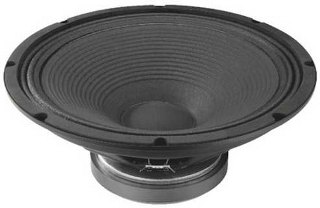open a bigger photo

recommended cabinet 1:
closed cabinet with 27 L volume
from 108/68 Hz (-3dB/-8dB)

recommended cabinet 2:
75 L volume bass reflex cabinet
with 2x HP100 reflex tube, 10 cm long.
from 61/47 Hz (-3dB/-8dB).

## Monacor SP-15/300PA

order no. mo-102540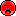- not available

37 cm bass driver with high quality cone.

• power handling (continuous/programme) = 300/600 W
• frequency range = 57-4500 Hz
• resonance frequency fs = 38 Hz
• impedance R = 8 Ohm
• sound pressure level SPL = 101 dB (2,83V; 1m)
• DC resistance Re = 6,8 Ohm
• force factor BL = 22,27 N/A
• voice coil inductance L = 1,3 mH
• effective piston radiating area Sd = 855 cm2
• effective mechanical mass incl. air load mms = 88 g
• equivalent volume of compliance Vas = 232 l
• total Q factor Qts = 0,28 (Qms=5,44, Qes=0,29)
• voice coil diameter = 78 mm
• maximum peak linear excursion vibration xlin = +/- 3,0 mm
• mounting diameter d = 358 mm
• overall diameter d = 388 mm
• mounting depth (not countersunk) t = 156 mm
• mass m = 7 kgopen a bigger photo

recommended cabinet 1:
closed cabinet with 13,4 L volume
from 107/68 Hz (-3dB/-8dB)

recommended cabinet 2:
28 L volume bass reflex cabinet
with HP100 reflex tube, 21 cm long.
from 68/50 Hz (-3dB/-8dB).

## Monacor FTR15-4080F

order no. mo-105050

- not available (_)

37 cm bass driver with high quality cone. To avoid compression effects the driver possesses a pole piece hole. The spider is rear ventilated for better cooling.

• power handling (continuous/programme) = 600/1200 W
• frequency range = 45-1500 Hz
• resonance frequency fs = 30 Hz
• impedance R = 8 Ohm
• sound pressure level SPL = 98 dB (2,83V; 1m)
• DC resistance Re = 5,5 Ohm
• force factor BL = 25,81 N/A
• voice coil inductance L = 1,5 mH
• effective piston radiating area Sd = 855 cm2
• effective mechanical mass incl. air load mms = 150 g
• equivalent volume of compliance Vas = 190 l
• total Q factor Qts = 0,22 (Qms=3,41, Qes=0,24)
• voice coil diameter = 100 mm
• maximum peak linear excursion vibration xlin = +/- 6,0 mm
• mounting diameter d = 352 mm
• overall diameter d = 388 mm
• mounting depth (not countersunk) t = 175 mm
• mass m = 9,4 kgrecommended cabinet 1:
closed cabinet with 93 L volume
from 65/41 Hz (-3dB/-8dB)

recommended cabinet 2:
372 L volume bass reflex cabinet
with 4x HP100 reflex tube, 8 cm long.
from 31/25 Hz (-3dB/-8dB).

## Monacor SPA-38PA

order no. mo-104050

- not available (_)

38 cm bass driver with paper cone. The basket is made of die-cast.

• power handling (continuous/programme) = 250/500 W
• frequency range = 55-3500 Hz
• resonance frequency fs = 37 Hz
• impedance R = 8 Ohm
• sound pressure level SPL = 98 dB (2,83V; 1m)
• DC resistance Re = 6 Ohm
• force factor BL = 14,32 N/A
• voice coil inductance L = 1 mH
• effective piston radiating area Sd = 855 cm2
• effective mechanical mass incl. air load mms = 80 g
• equivalent volume of compliance Vas = 230 l
• total Q factor Qts = 0,45 (Qms=2,54, Qes=0,55)
• voice coil diameter = 65 mm
• maximum peak linear excursion vibration xlin = +/- 3 mm
• mounting diameter d = 354 mm
• overall diameter d = 402 mm
• mounting depth (not countersunk) t = 138 mmrecommended cabinet 1:
closed cabinet with 35 L volume
from 103/65 Hz (-3dB/-8dB)

recommended cabinet 2:
128 L volume bass reflex cabinet
with 2x HP100 reflex tube, 3 cm long.
from 52/41 Hz (-3dB/-8dB).

## Monacor SP-38A/300PRO

order no. mo-104530

- not available (_)

37 cm bass driver with high quality cone. The basket is made of die-cast.

• power handling (continuous/programme) = 300/600 W
• frequency range = 70-4000 Hz
• resonance frequency fs = 50 Hz
• impedance R = 8 Ohm
• sound pressure level SPL = 102 dB (2,83V; 1m)
• DC resistance Re = 6 Ohm
• force factor BL = 18,54 N/A
• voice coil inductance L = 0,85 mH
• effective piston radiating area Sd = 880 cm2
• effective mechanical mass incl. air load mms = 80 g
• equivalent volume of compliance Vas = 135 l
• total Q factor Qts = 0,39 (Qms=3,37, Qes=0,44)
• voice coil diameter = 75 mm
• maximum peak linear excursion vibration xlin = +/- 2,5 mm
• mounting diameter d = 354 mm
• overall diameter d = 392 mm
• mounting depth (not countersunk) t = 149 mmopen a bigger photo

recommended cabinet 1:
closed cabinet with 36 L volume
from 82/52 Hz (-3dB/-8dB)

recommended cabinet 2:
85 L volume bass reflex cabinet
with 2x HP100 reflex tube, 20 cm long.
from 50/37 Hz (-3dB/-8dB).

## Monacor FTR18-4080F

order no. mo-105060

- not available (_)

44 cm bass driver with high quality cone. To avoid compression effects the driver possesses a pole piece hole. The spider is rear ventilated for better cooling.

• power handling (continuous/programme) = 600-1200 W
• frequency range = 37-500 Hz
• resonance frequency fs = 25 Hz
• impedance R = 8 Ohm
• sound pressure level SPL = 99 dB (2,83V; 1m)
• DC resistance Re = 5,6 Ohm
• force factor BL = 24,12 N/A
• voice coil inductance L = 1,5 mH
• effective piston radiating area Sd = 1164 cm2
• effective mechanical mass incl. air load mms = 169 g
• equivalent volume of compliance Vas = 430 l
• total Q factor Qts = 0,24 (Qms=3,78, Qes=0,26)
• voice coil diameter = 100 mm
• maximum peak linear excursion vibration xlin = +/- 6,0 mm
• mounting diameter d = 414 mm
• overall diameter d = 453 mm
• mounting depth (not countersunk) t = 206 mm
• mass m = 9,7 kg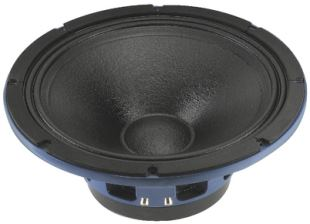recommended cabinet 1:
closed cabinet with 26 L volume
from 101/64 Hz (-3dB/-8dB)

recommended cabinet 2:
64 L volume bass reflex cabinet
with 2x HP100 reflex tube, 16 cm long.
from 60/45 Hz (-3dB/-8dB).

## Monacor SP-46A/500PA

order no. mo-103420

- not available (_)

45 cm bass driver with paper cone. The basket is made of die-cast.

• power handling (continuous/programme) = 500/2000 W
• frequency range = 48-2500 Hz
• resonance frequency fs = 32 Hz
• impedance R = 8 Ohm
• sound pressure level SPL = 99 dB (2,83V; 1m)
• DC resistance Re = 6,4 Ohm
• force factor BL = 29,1 N/A
• voice coil inductance L = 1,7 mH
• effective piston radiating area Sd = 1180 cm2
• effective mechanical mass incl. air load mms = 168 g
• equivalent volume of compliance Vas = 285 l
• total Q factor Qts = 0,25 (Qms=6,65, Qes=0,26)
• voice coil diameter = 99 mm
• maximum peak linear excursion vibration xlin = +/- 6 mm
• mounting diameter d = 416 mm
• overall diameter d = 470 mm
• mounting depth (not countersunk) t = 194 mm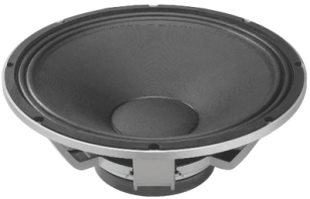recommended cabinet 1:
closed cabinet with 177 L volume
from 51/32 Hz (-3dB/-8dB)

recommended cabinet 2:
712 L volume bass reflex cabinet
with 4x HP100 reflex tube, 6 cm long.
from 24/20 Hz (-3dB/-8dB).

## Monacor SPA-45PA

order no. mo-104060

- not available (_)

45 cm bass driver with paper cone. The basket is made of die-cast.

• power handling (continuous/programme) = 300/600 W
• frequency range = 44-3500 Hz
• resonance frequency fs = 29 Hz
• impedance R = 8 Ohm
• sound pressure level SPL = 98 dB (2,83V; 1m)
• DC resistance Re = 5,8 Ohm
• force factor BL = 16,91 N/A
• voice coil inductance L = 1 mH
• effective piston radiating area Sd = 1225 cm2
• effective mechanical mass incl. air load mms = 136 g
• equivalent volume of compliance Vas = 440 l
• total Q factor Qts = 0,46 (Qms=3,77, Qes=0,52)
• voice coil diameter = 75 mm
• maximum peak linear excursion vibration xlin = +/- 2 mm
• mounting diameter d = 424 mm
• overall diameter d = 464 mm
• mounting depth (not countersunk) t = 159 mm

The cabinet volume calculations are executed considering an external resistance of 0.3 Ohm. We are using, if no other data is known or available, the information supplied by the manufacturer.

Please note: the frequency response doesn't say much about the cut-off frequency inside the cabinet! Therefore, we mostly give you 2 examples with dimensioning considerations and an indication, how low the loudspeaker will reproduce.

Home